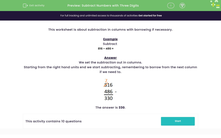# Subtract Numbers with Three Digits

In this worksheet, students subtract numbers with three digits using the column method with borrowing if necessary.Key stage:  KS 2

Curriculum topic:   Number: Addition and Subtraction

Curriculum subtopic:   Add/Subtract up to Four-Digit Numbers

Difficulty level:#### Worksheet Overview

This worksheet is about subtraction in columns with borrowing if necessary.

Example

Subtract

816 – 486 =

We set the subtraction out in columns.

Starting from the right hand units end we start subtracting, remembering to borrow from the next column if we need to.